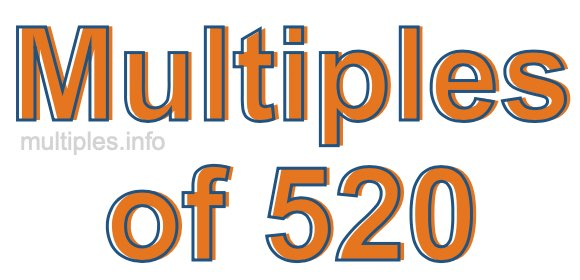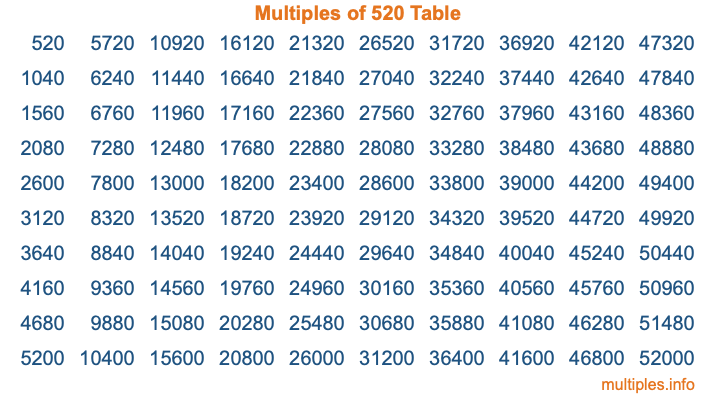Multiples of 520Welcome to the Multiples of 520 page. Here we will first teach you everything you will ever need to know about the multiples of 520, and then give you a study guide summary of everything we taught you to make sure you remember it all. Use this page to look up facts and learn information about the multiples of 520. This page will make you a multiples of five hundred twenty expert!

Definition of Multiples of 520
Multiples of 520 are all the numbers that when divided by 520 equal an integer. Each of the multiples of 520 are called a multiple. A multiple of 520 is created by multiplying 520 by an integer.

Therefore, to create a list of multiples of 520, you start with 1 multiplied by 520, then 2 multiplied by 520, then 3 multiplied by 520, and so on for as long as you want. Thus, the list of the first five multiples of 520 is 520, 1040, 1560, 2080, and 2600. To see a larger list of multiples of 520, see the printable image of Multiples of 520 further down on this page. We also have a category where you can choose any nth multiple of 520.

Multiples of 520 Checker
The Multiples of 520 Checker below checks to see if any number of your choice is a multiple of 520. In other words, it checks to see if there is any number (integer) that when multiplied by 520 will equal your number. To do that, we divide your number by 520. If the the quotient is an integer, then your number is a multiple of 520.

Is  a multiple of 520?

Least Common Multiple of 520 and ...
A Least Common Multiple (LCM) is the lowest multiple that two or more numbers have in common. This is also called the smallest common multiple or lowest common multiple and is useful to know when you are adding our subtracting fractions. Enter one or more numbers below (520 is already entered) to find the LCM.

Check out our LCM Calculator if you need more details about the Least Common Multiple or if you need the LCM for different numbers for adding and subtraction fractions.

nth Multiple of 520
As we stated above, 520 is the first multiple of 520, 1040 is the second multiple of 520, 1560 is the third multiple of 520, and so on. Enter a number below to find the nth multiple of 520.

th multiple of 520

Multiples of 520 vs Factors of 520
520 is a multiple of 520 and a factor of 520, but that is where the similarities end. All postive multiples of 520 are 520 or greater than 520. All positive factors of 520 are 520 or less than 520.

Below is the beginning list of multiples of 520 and the factors of 520 so you can compare:

Multiples of 520: 520, 1040, 1560, 2080, 2600, etc.

Factors of 520: 1, 2, 4, 5, 8, 10, 13, 20, 26, 40, 52, 65, 104, 130, 260, 520

As you can see, the multiples of 520 are all the numbers that you can divide by 520 to get a whole number. The factors of 520, on the other hand, are all the whole numbers that you can multiply by another whole number to get 520.

It's also interesting to note that if a number (x) is a factor of 520, then 520 will also be a multiple of that number (x).

Multiples of 520 vs Divisors of 520
The divisors of 520 are all the integers that 520 can be divided by evenly. Below is a list of the divisors of 520.

Divisors of 520: 1, 2, 4, 5, 8, 10, 13, 20, 26, 40, 52, 65, 104, 130, 260, 520

The interesting thing to note here is that if you take any multiple of 520 and divide it by a divisor of 520, you will see that the quotient is an integer.

Multiples of 520 Table
Below is an image of the first 100 multiples of 520 in a table. The table is in chronological order, column by column. The first column has the first ten multiples of 520, the second column has the next ten multiples of 520, and so on.The Multiples of 520 Table is also referred to as the 520 Times Table or Times Table of 520. You are welcome to print out our table for your studies.

Negative Multiples of 520
Although not often discussed or needed in math, it is worth mentioning that you can make a list of negative multiples of 520 by multiplying 520 by -1, then by -2, then by -3, and so on, to get the following list of negative multiples of 520:

-520, -1040, -1560, -2080, -2600, etc.

Multiples of 520 Summary
Below is a summary of important Multiples of 520 facts that we have discussed on this page. To retain the knowledge on this page, we recommend that you read through the summary and explain to yourself or a study partner why they hold true.

There are an infinite number of multiples of 520.

A multiple of 520 divided by 520 will equal a whole number.

520 divided by a factor of 520 equals a divisor of 520.

The nth multiple of 520 is n times 520.

The largest factor of 520 is equal to the first positive multiple of 520.

520 is a multiple of every factor of 520.

520 is a multiple of 520.

A multiple of 520 divided by a divisor of 520 equals an integer.

520 divided by a divisor of 520 equals a factor of 520.

Any integer times 520 will equal a multiple of 520.

Multiples of a Number
Here you can get the multiples of another number, all with the same attention to detail as we did for multiples of 520 on this page.

Multiples of
Multiples of 521
Did you find our page about multiples of five hundred twenty educational? Do you want more knowledge? Check out the multiples of the next number on our list!